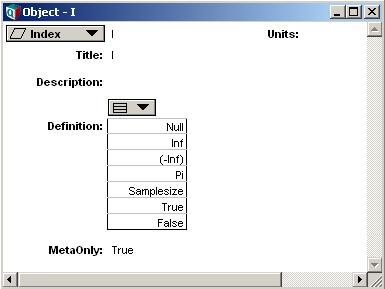# MetaOnly

## Attribute MetaOnly

When this attribute is set to True for an Index object, associative lookup (A[I = x]) or @[I = x]) does not evaluate the index elements, and associative lookup matches only to the raw unevaluated index elements.

This alters the behavior of associative lookup when index items consist of object identifiers (i.e., handles), or expressions. This alteration in behavior is often needed when performing Meta-Inference, that is, algorithms that reason about or alter the objects and structure of the model itself. Meta-Inference is an advanced Analytica modeling topic.

## Example

Consider a model with the following objects, defined as shown:

Constant E := Exp(1)
Variable A := 1 + 2
Variable B := 7
Index J := [E, A, B]
Variable T := Table(J)(11, 12, 13)

With these definitions, consider the following subscript expressions:

T[J = E] → 11
T[J = 3] → 12
T[J = 7] → 13
T[J = B] → 13
T[J = Handle(E)] → 11
T[J = Handle(A)] → 12

Normally, all of the above subscript operations succeed. However, in order for Analytica to recognize that T[J = 3] identifies the second element of J, T[@J = 2], Analytica must evaluate the variables (or expressions) that are listed in the definition of J. If any of these evaluate to an atom, then the atom is recognized by associative lookup. But to know this, Analytica must evaluate all of the elements of the index.

When performing Meta-Inference, this flexibility may be undesirable, and may cause problems that prevent you from doing what you want to do. For example, if a variable found in the index is not yet defined, or otherwise has an error in its definition, an evaluation error might result when you attempt to subscript on J. A variable listed may perform a very complex computation requiring hours to evaluate, the result of which is irrelevant to your meta-inference algorithm. Or, a variable might evaluate to a Handle and create an ambiguity for subscript. In each of these cases, in order to perform meta-inference without these complications, you can set the MetaOnly attribute for the Index to True.

In the above example, suppose we set the MetaOnly attribute of J to True. Now the variables listed in J's definition are not evaluated when you subscript on J, and only elements directly appearing as elements in J match, in this case, only the handles. The above evaluates are now (where Null indicates the element is not found):

T[J = E] → Null
T[J = 3] → Null
T[J = 7] → Null
T[J = B] → Null
T[J = Handle(E)] → 11
T[J = Handle(A)] → 12

Suppose modify the definition of B to be:

Variable B := Bernoulli(-1)

This is an error that prevents B from being evaluated. In the case where MetaOnly is false, no subscript operation on J is possible -- any attempt to subscript over J will fail with the error when evaluating B. However, your meta-inference algorithm may be dealing with node layout, navigation, etc., having nothing to do with the value of B. By setting MetaOnly of J to True, the error in B does not prevent your meta-inference algorithm from functioning.

When you are using a local index for meta-inference, you can declare the local index with the MetaIndex..Do construct, instead of the Index..Do construct, which has the effect of setting the MetaOnly attribute implicitly. However, for global indexes (those appearing on a diagram), your only method for getting the MetaOnly behavior is by setting the attribute value.

## Caveats

If you set the MetaOnly attribute on an index object (or use the LocalIndex..Do declaration to define a local index), you should be aware that associative indexing will not find certain system variable constants in the form you might expect. For example, if a global index is defined as a list with the MetaOnly attribute set:Then these subscript operations won't find the value:

@[I = Null] → 0 {not found}
@[I = Inf] → 0
@[I = Pi] → 0
@[I = True] → 0

The values can be located using:

@[I = Handle(Null)] → 1
@[I = Handle(Inf)] → 2
@[I = Handle(Pi)] → 4
@[I = Handle(True)] → 6

## History

MetaOnly attribute was introduced in Analytica 4.0, it is not present prior to release 4.0.0.35.